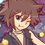# Functional Equation (Thailand Math POSN 2nd round)

Write a full solution.

1.) Find all functions $f: \mathbb{N} \rightarrow \mathbb{N}$ that satisfy the inequality for all $n \in \mathbb{N}$

$f(n+1) > f(f(n))$

2.) Let $f: \mathbb{N} \rightarrow \mathbb{N}$ be a function that satisfy the equation for all $m,n \in \mathbb{N}$

$f(m+f(n)) = n+f(m+58)$

Prove that there exists only 1 solution of functional equation above, and find the value of $f(1)+f(2)+\dots + f(15)$.

3.) Find all functions $f: \mathbb{R_{0}^{+}} \rightarrow \mathbb{R_{0}^{+}}$ such that for all $x,y \in \mathbb{R_{0}^{+}}$

$f(x+f(y^{2})) = f(x) + y^{2}$

Where $\mathbb{R_{0}^{+}}$ is set of non-negative real numbers.

4.) Find all functions $f: \mathbb{R} \rightarrow \mathbb{R}$ such that for all $x,y,z \in \mathbb{R}$

$f(x^{2}(z^{2}+1)+f(y)(z+1)) = 1 - f(z)(x^{2}+f(y)) - z((1+z)x^{2}+2f(y))$

5.) Let $f: \mathbb{R} \rightarrow \mathbb{R}$ be a function that satisfy the equation for all $x,y \in \mathbb{R}$

$f^{3}(x+y)+f^{3}(x-y) = (f(x)+f(y))^{3}+(f(x)-f(y))^{3}$

Prove that $f(x+y) = f(x)+f(y)$ for all $x,y \in \mathbb{R}$.

This note is a part of Thailand Math POSN 2nd round 2015Note by Samuraiwarm Tsunayoshi
6 years, 2 months ago

This discussion board is a place to discuss our Daily Challenges and the math and science related to those challenges. Explanations are more than just a solution — they should explain the steps and thinking strategies that you used to obtain the solution. Comments should further the discussion of math and science.

When posting on Brilliant:

• Use the emojis to react to an explanation, whether you're congratulating a job well done , or just really confused .
• Ask specific questions about the challenge or the steps in somebody's explanation. Well-posed questions can add a lot to the discussion, but posting "I don't understand!" doesn't help anyone.
• Try to contribute something new to the discussion, whether it is an extension, generalization or other idea related to the challenge.
• Stay on topic — we're all here to learn more about math and science, not to hear about your favorite get-rich-quick scheme or current world events.

MarkdownAppears as
*italics* or _italics_ italics
**bold** or __bold__ bold
- bulleted- list
• bulleted
• list
1. numbered2. list
1. numbered
2. list
Note: you must add a full line of space before and after lists for them to show up correctly
paragraph 1paragraph 2

paragraph 1

paragraph 2

[example link](https://brilliant.org)example link
> This is a quote
This is a quote
    # I indented these lines
# 4 spaces, and now they show
# up as a code block.

print "hello world"
# I indented these lines
# 4 spaces, and now they show
# up as a code block.

print "hello world"
MathAppears as
Remember to wrap math in $$ ... $$ or $ ... $ to ensure proper formatting.
2 \times 3 $2 \times 3$
2^{34} $2^{34}$
a_{i-1} $a_{i-1}$
\frac{2}{3} $\frac{2}{3}$
\sqrt{2} $\sqrt{2}$
\sum_{i=1}^3 $\sum_{i=1}^3$
\sin \theta $\sin \theta$
\boxed{123} $\boxed{123}$

## Comments

Sort by:

Top Newest

Samuraiwarm Tsunayoshi, I wanted to ask that for Q-5, can we use a particular $f(x)$ that satisfies the given condition to prove the required statement?

If so, then one can note that the class of functions $f:\Bbb{R}\mapsto\Bbb{R}$ defined by $f(x)=\lambda x~,~\lambda$ is some constant satisfies the given condition. Then, the proof becomes trivial since,

$f(x+y)=\lambda (x+y)=\lambda x + \lambda y = f(x)+f(y)$

- 6 years, 2 months ago

Log in to reply

Nope, you have to prove that no other functions exist.

- 6 years, 2 months ago

Log in to reply

×

Problem Loading...

Note Loading...

Set Loading...